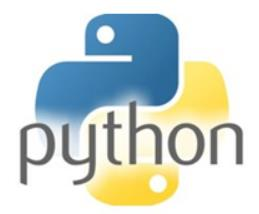## 每个Python数据分析师都应掌握的10个Pandas基本技巧

Excel中的Python：将重塑数据分析师的工作方式
5个超棒的Python项目！

1 重新命名列

``````import pandas as pd

# Create a sample DataFrame
data = {'old_name_1': [1, 2, 3],
'old_name_2': [4, 5, 6]}
df = pd.DataFrame(data)
# Rename columns
df.rename(columns={'old_name_1': 'new_name_1', 'old_name_2': 'new_name_2'}, inplace=True)``````

2 按条件筛选行

``````# Filter rows where a condition is met
filtered_df = df[df['column_name'] > 3]``````

3 处理缺失数据

``````# Drop rows with missing values
df.dropna()

# Fill missing values with a specific value
df.fillna(0)``````

4 分组和汇总数据

``````# Group by a column and calculate mean for each group
grouped = df.groupby('group_column')['value_column'].mean()``````

5 透视表

``````# Create a pivot table
pivot_table = df.pivot_table(values='value_column', index='row_column', columns='column_column', aggfunc='mean')``````

6 合并数据框

``````# Merge two DataFrames
merged_df = pd.merge(df1, df2, on='common_column', how='inner')``````

7 应用自定义功能

``````# Apply a custom function to a column
def custom_function(x):
return x * 2

df['new_column'] = df['old_column'].apply(custom_function)``````

8 对时间序列数据重新取样

``````# Resample time series data
df['date_column'] = pd.to_datetime(df['date_column'])
df.resample('D', on='date_column').mean()``````

9 处理分类数据

``````# Convert categorical data to numerical using one-hot encoding
df = pd.get_dummies(df, columns=['categorical_column'])``````

10 导出数据

``````# Export DataFrame to CSV
df.to_csv('output.csv', index=False)``````